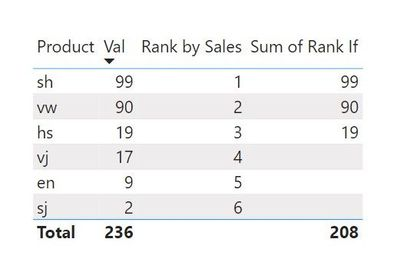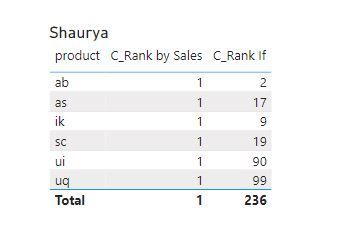cancel
Showing results for
Did you mean:New Member

## RANK, TOP N - CORRECT SUM IN THE TABLE

Hi All,

Please help me with the correct sum in the table. While using rank I would like to see sum only for selected products. Thx.1 ACCEPTED SOLUTIONNew Member

I have managed with it already by such measure:

CORRECT SUM = if(int([HAS_ONE_VALUE_?])=1,[RANK IF],SUMX(VALUES(Table1[product]),[RANK IF]))
4 REPLIES 4New Member

I have managed with it already by such measure:

CORRECT SUM = if(int([HAS_ONE_VALUE_?])=1,[RANK IF],SUMX(VALUES(Table1[product]),[RANK IF]))Memorable Member

Hi @termini,

I am not exactly sure why you are using the TopN table but I used these formulas to replicate your data:

``Rank by Sales = RANKX('Table','Table'[Value],,DESC)``

``Rank If = IF('Table'[Rank by Sales]<=3,'Table'[Value])``

I used these two along with the Product and Value column and got the right total.Works for you? Mark this post as a solution if it does!
Consider taking a look at my blog: Forecast Period - Previous ForecastsNew Member

unfortunatelly this measure

`Rank by Sales = RANKX('Table','Table'[Value],,DESC)`

gives me rank of 1 in each line (for each product)Community Support

Hi , @termini

For your rankx , you need to use this dax :

``Rank by Sales= RANKX( ALL('Table'[product]) ,CALCULATE( SUM('Table'[val])),,DESC)``

Best Regards,

Aniya Zhang

If this post helps, then please consider Accept it as the solution to help the other members find it more quickly